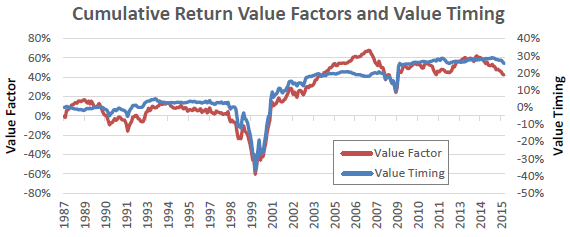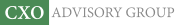Objective research to aid investing decisions

#### Value Investing Strategy (Strategy Overview)

Allocations for October 2023 (Final)
Cash TLT LQD SPY

#### Momentum Investing Strategy (Strategy Overview)

Allocations for October 2023 (Final)
1st ETF 2nd ETF 3rd ETF

# Factor Portfolio Valuation and Timing of Factor Premiums

| | Posted in: Momentum Investing, Value Premium

Does timing of factor premiums work? In his June 2016 paper entitled “My Factor Philippic”, Clifford Asness addresses three critiques of the exploitability of stock factor premiums:

1. Most factors are currently very overvalued (expected premiums are small), perhaps because of crowded bets on them.
2. Factor portfolios may therefore crash.
3. In fact, increasing factor valuations account for most of the historical premium (there are no essential premiums).

He considers five long-short factors: (1) value based on book-to-price ratio (B/P); (2) value based on sales-to-price ratio (S/P); (3) momentum (total return from 12 months ago to one month ago); (4) profitability (gross profits-to-assets); and, (5) betting-against-beta (long leveraged low-beta assets and short high-beta assets). He calculates each factor premium as average return to a capitalization-weighted portfolio that is each month long (short) the third of large-capitalization U.S. stocks with the best (worst) expected returns based on that factor. He estimates the time-varying valuation of a factor via a value spread, the ratio of the capitalization-weighted B/P (or S/P) of the long side of the factor portfolio to that of its short side. He tests a simple factor timing strategy that holds no position if the factor’s value spread is at its historical median and scales linearly up (down) to a 100% (-100%) position in the factor portfolio as the factor’s value spread increases to its 95th (decreases to its 5th) historical percentile. The initial look-back interval is 20 years (such that testing begins in 1988), expanding as more data become available. Using the specified factor premium data for January 1968 through January 2016, he finds that:

• Based on the specified value spread approach as of January 2016:
• The two value factors appear somewhat undervalued.
• The profitability factor appears somewhat overvalued.
• Momentum and betting-on-beta factors appear significantly overvalued, but arguably not in bubble states.
• Tactical-horizon regressions of future returns on factor value spreads suggest weak return predictability based on factor valuations, but associated factor timing strategies add little to portfolios already exploiting the value factor (see the chart below).
• Factor value spread trends generally account for little of the respective long-term factor premiums.

The following chart, taken from the paper, compares gross cumulative average excess returns of:

• Value Factor – average of the two simple long-short value factors based on B/P or S/P.
• Value Timing – average of the 10 timing strategies as specified above for the five factors with value spreads measured either via B/P or S/P.

Results from timing the factors based on value spreads are little different from simple value factor exposure. Findings are robust to the exact timing methodology and to the combination of factors timed.In summary, evidence mostly supports beliefs that: (1) factor premiums are not extremely overvalued; (2) timing of stock factor premiums based on value spreads is ineffective; and, (2) factor valuation changes do not account for historical factor premiums.

Cautions regarding findings include:

• Factor premium calculations are gross, not net. Accounting for costs of portfolio turnover and shorting would reduce premiums. These costs likely affect some factors more than others.
• Stock factor portfolio construction/implementation is beyond the reach of many investors, who would bear fees for accessing associated premiums via fund or investment manager.
• It is not obvious that the “value spread” approach, which ties timing of all factors inherently to the value premium, is the most logical basis for factor timing. Other approaches might use momentum or reversal of a rolling factor premium.